Courses

# Test: Types of Fluids

## 10 Questions MCQ Test Fluid Mechanics | Test: Types of Fluids

Description
This mock test of Test: Types of Fluids for Civil Engineering (CE) helps you for every Civil Engineering (CE) entrance exam. This contains 10 Multiple Choice Questions for Civil Engineering (CE) Test: Types of Fluids (mcq) to study with solutions a complete question bank. The solved questions answers in this Test: Types of Fluids quiz give you a good mix of easy questions and tough questions. Civil Engineering (CE) students definitely take this Test: Types of Fluids exercise for a better result in the exam. You can find other Test: Types of Fluids extra questions, long questions & short questions for Civil Engineering (CE) on EduRev as well by searching above.
QUESTION: 1

### The relation between shear stress Z and velocity gradient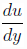of a fluid is given by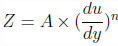​ where A and n are constants. If n = 1, what type of fluid will it be?

Solution:

Explanation: When n = 1, the relation reduces to Newton’s law of viscosity: z = A *, where A will represent the viscosity of the fluid. The fluid following this relation will be a Newtonian fluid.

QUESTION: 2

### The relation between shear stress Z and velocity gradientof a fluid is given by​  where A and n are constants. What type of fluid will it be if n < 1 and n > 1 respectively?

Solution:

Explanation: When n ≠ 1, the relation will be treated as Power law for Non-Newtonian fluids:. For n < 1, the rate of change of the shear stress decreases with the increase in the value of velocity gradient. Such fluids are called Pseudoplastics.

For n > 1, the rate of change of the shear stress increases with the increase in the value of velocity gradient. Such fluids are called Dilatants.

QUESTION: 3

###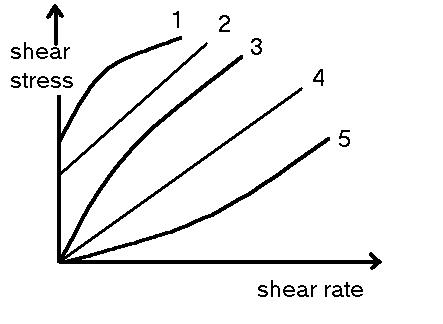In the above graph, what types of fluids are represented by the lines 2 and 3 respectively ?

Solution:
QUESTION: 4

The relation between shear stress Z and velocity gradientof a fluid is given by​  + B where A, n and B are constants.
Which of the following conditions will hold for a Bingham plastic?

Solution:

Explanation: For Bingham Plastics, shear stress will not remain constant after an yield value of stress. Thus, A ≠ 0;B ≠ 0. After the yield value, the relation between the shear stress and velocity gradient will become linear. hus, n = 1.

QUESTION: 5

The relation between shear stress Z and velocity gradientof a fluid is given by​  + B where A, n and B are constants. Which of the following conditions will hold for a Rheopectic?

Solution:

Explanation: For Rheopectics, shear stress will not remain constant after an yield value of stress. Thus, A ≠ 0; B ≠ 0. After the yield value, the rate of change of the shear stress increases with the increase in the value of velocity gradient. Thus, n > 1.

QUESTION: 6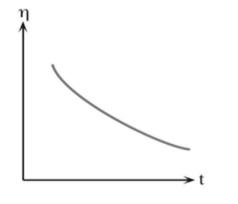The above graph of viscosity vs time depicts which of the following fluids?

(Hint : This fluid is present in inks and paints)

Solution:

Explanation: For Thixotropics,their viscosity is time dependent and decreases as time goes on.

QUESTION: 7

The relation between shear stress Z and velocity gradientof a fluid is given by​ where A and n are constants. The graphs are drawn for three values of n. Which one will be the correct relationship between n1, n2 and n3?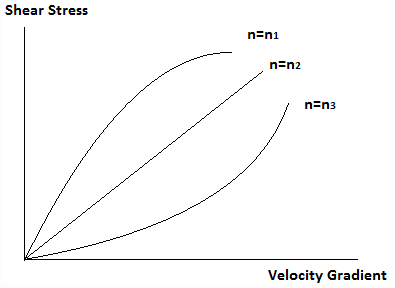Solution:

Explanation: The graph corresponding to n = n1 represents Pseudoplastics, for which the rate of change of the shear stress decreases with the increase in the value of velocity gradient. The graph corresponding to n = n2 represents Newtonian fluids, for which shear stress changes linearly with the change in velocity gradient. The graph corresponding to n = n3 represents Dilatents, for which the rate of change of the shear stress increases with the increase in the value of velocity gradient.

QUESTION: 8

Which of the following is a shear-thinnning fluid?

Solution:

Explanation: Shear-thinning fluids are those which gets strained easily at high values of shear stresses. The relation between shear stress Z and velocity gradientof a shear-thinning fluid is given by, where A and n are constants and n < 1. This relation is followed by Pseudoplastics.

QUESTION: 9

Which of the following is a shear-thickening fluid?

Solution:

Explanation: Shear-thickening fluids are those for which it gets harger to strain it at high values of shear stresses. The relation between shear stress Z and velocity gradientof a shear-thickening fluid is given bywhere A and n are constants and n > 1. This relation is followed by Dilatants.

QUESTION: 10

What will be the dimension of the flow consistency index for a fluid with a flow behaviour index of -1?

Solution:

Explanation: The relation between shear stress Z and velocity gradientof a fluid is given bywhere A is the flow consistency index and n is the flow behaviour index. If n = -1, A = Z *Unit of Z is N/m2 andis s-1. Thus, the unit of A will be N/m2 s.

Track your progress, build streaks, highlight & save important lessons and more!

### Similar Content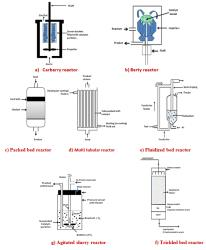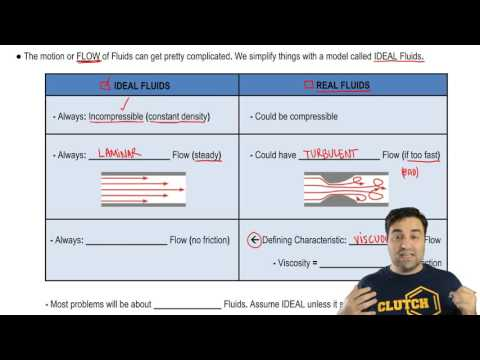### Related tests